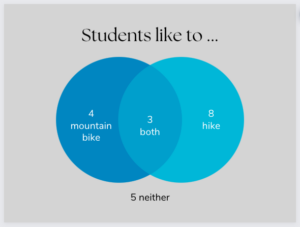Part 10: Data Analysis and Probability

# 10.2: Calculating probabilities

Notes

Simply put, probabilities are numbers between 0 and 1 that represent the chance of some outcome occurring. For example, suppose that out of a class of 20 students:

• 9 take the bus to school
• 6 ride their bike to school
• 5 walk to school
##### Select one student at random
• Since 9 students out of 20 take the bus, the probability that the selected student takes the bus to school is 9/20 = 0.45 = 45%.
• Since 6 students out of 20 ride their bike, the probability that the selected student rides their bike to school is 6/20 = 0.3 = 30%.
• Since 5 students out of 20 walk, the probability that the selected student walks to school is 5/20 = 0.25 = 25%.
• Note that the probabilities sum to 1: 0.45 + 0.3 + 0.25 = 1.
• What is the probability that the selected student either takes the bus or walks to school?
• The probability that the selected student takes the bus to school is 9/20.
• The probability that the selected student walks to school is 5/20.
• Add these two probabilities to find the probability that the selected student either takes the bus or walks to school: 9/20 + 5/20 = 14/20 = 7/10 = 0.7 = 70%.
##### Select two students at random without replacement

Here, we’re not replacing the first selected student in the list of potential students to select from before selecting the second student. (Think of selecting the students on the same day.)

• What is the probability that both students ride their bike to school?
• The probability that the first selected student rides their bike to school is 6/20.
• Since there are now just 19 students to select from and only 5 of them ride their bike to school, the probability that the second selected student rides their bike to school is 5/19.
• Multiply these two probabilities to find the probability that both students ride their bike to school: 6/20 x 5/19 = 3/38 = 0.07895 = 7.895%.
##### Select two students at random with replacement

Here, we are replacing the first selected student in the list of potential students to select from before selecting the second student. (Think of selecting one student on Monday and the other student on Tuesday.)

• What is the probability that both students ride their bike to school?
• The probability that the first selected student rides their bike to school is 6/20.
• The probability that the second selected student rides their bike to school is also 6/20.
• Multiply these two probabilities to find the probability that both students ride their bike to school: 6/20 x 6/20 = 9/100 = 0.09 = 9%.
##### Working with two categories

For example, suppose that out of a class of 20 students:

• 7 like to mountain bike
• 11 like to hike
• 3 like to do both (mountain bike and hike)

Since 7 students like to mountain bike and 3 students like to both mountain bike and hike, there must be 7 – 3 = 4 students who like to mountain bike but don’t like to hike.

Similarly, 11 students like to hike and 3 students like to both mountain bike and hike, so there must be 11 – 3 = 8 students who like to hike but don’t like to mountain bike.

How many students don’t like either mountain biking or hiking? We’ve accounted for 4 students who like to mountain bike but don’t like to hike, 8 students who like to hike but don’t like to mountain bike, and 3 students who like to both mountain bike and hike. Therefore, there must be 20 – 4 – 8 – 3 = 5 students left who don’t like either mountain biking or hiking.

We can summarize all this information in a Venn diagram:We can also calculate some probabilities based on selecting a student at random from this class:

• What is the probability that the selected student likes to both mountain bike and hike? 3/20 = 0.15 = 15%.
• What is the probability that the selected student likes to mountain bike but doesn’t like to hike? Calculate this as 7/20 – 3/20 = 4/20 = 0.2 = 20%.
• What is the probability that the selected student likes to mountain bike or hike (or both)? Calculate this as (7/20 – 3/20) + (11/20 – 3/20) + 3/20 = 4/20 + 8/20 + 3/20 = 15/20 = 0.75 = 75%.
• Note that this last probability can also be calculated as 7/20 + 11/20 – 3/20 = 15/20 = 0.75 = 75%.

The videos below work through some examples of calculating probabilities.

Practice Exercises

Do the following exercises to practice calculating probabilities.

In a collection of 25 dogs, 10 are large dogs with long hair, 3 are large dogs with short hair, 5 are small dogs with long hair, and 7 are small dogs with short hair.